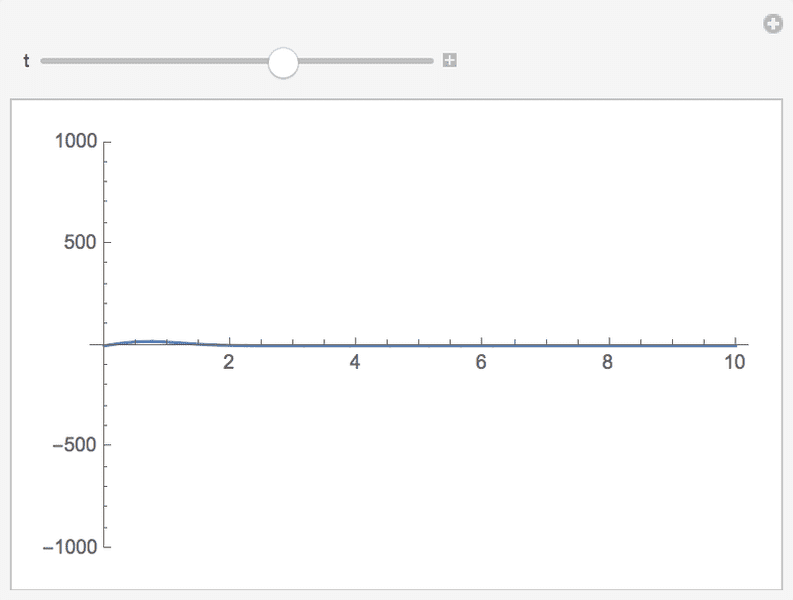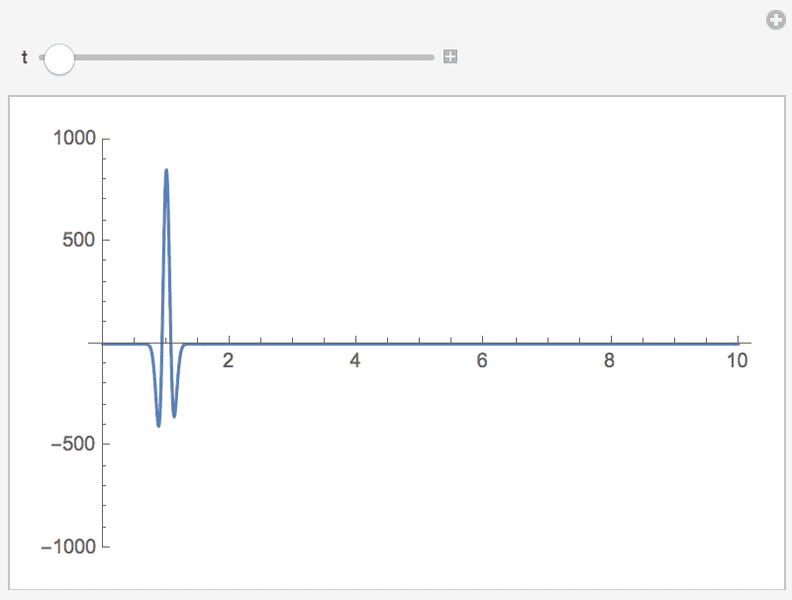# Verifying PDE solutions using Mathematica

• Mathematica
Hello physicists,

Pretty new to Mathematica here. I'm looking to verify that $$P(s,\tilde{t}|_{s_0}) = 2\tilde{b}_{\rho} \frac{s^{\alpha+1}}{\check{s_0}^{\alpha}}I_{\alpha}(2\tilde{b}_{\rho}s\check{s}_0)exp[-\tilde{b}_{\rho}(s^2+\check{s_0}^2)]$$
Is a solution to
$$\frac{\partial}{\partial{\tilde{t}}}P(s, \tilde{t}) = \frac{\partial}{\partial s}[(2b_{\rho}s - \frac{\rho}{s})P(s,\tilde{t})] + \frac{\partial^2}{\partial s^2}[P(s,\tilde{t})]$$

Where $$\alpha = \frac{\rho - 1}{2}$$ $$\tilde{b}_{\rho} = \frac{b_{\rho}}{1-e^{-\tilde{t}}}$$ $$\check{s}_0 = s_0 exp(\frac{-\tilde{t}}{2})$$ and $$I_{\alpha}$$ is a modified Bessel function of the first kind.

Following with this link, I wrote the following into Mathematica:

First I defined the variables in the solution as given above:

a = (r - 1)/2

b = b1/(1 - Exp[-t])

s0 = s01*Exp[-t/2]

i = BesselI[a, 2*b*s*s0]

I entered the solution like such:

q[s, t] = 2 b s^(a + 1)/(s0)*i*Exp[-b (s^2 + s0^2)]

I entered the general form of the equation:

E3 = D[p[s, t], t] == D[(2 b1 s - r/s) p[s, t], s] + D[p[s, t], {s, 2}]

Now I'm looking to "replace" the proposed solution q into E3 as p[s,t] and hope to get {True} as the output:

Simplify[E3 /. q[s, t]]

But the output is says: "___[contents of q]__ is neither a list of replacement rules nor a valid dispatch table and so cannot be used for replacing."

So I must be assigning something incorrectly... Does you see something wrong with this or know of an easier way to verify PDE solutions using Mathematica?

Thanks!

A replacement needs so-called rules. https://reference.wolfram.com/language/ref/Rule.html

There is a more straightforward way though.
Instead of defining a variable E3 containing
Code:
D[p[s, t], t] == D[(2 b1 s - r/s) p[s, t], s] + D[p[s, t], {s, 2}]

You simply write
Code:
Simplify[ D[q[s, t], t] == D[(2 b1 s - r/s) q[s, t], s] + D[q[s, t], {s, 2}] ]

That is you directly use your definition of the full solution.
I'm not sure if it's useful to write ##q[s,t]## if you're not using it further on.
Also ensure that you know the difference between
Code:
q[s,t] = ...
q[s,t] := ...
q[s_ , t_] := ...

Finally you can also use Reduce instead of Simplify which might be faster in more complicated cases.

I've ended up substituting the solution into the equation and subtracting one side from the other, hopefully to get zero.
Code:
p[s_, t_] = 2 b s^(a + 1)/(s0)*i*Exp[-b (s^2 + s0^2)]
E3 = D[p[s, t], t]
E4 = D[(2 b1 s - r/s) p[s, t], s] + D[p[s, t], {s, 2}]
expr = Simplify[E3 - E4]
E6 = Simplify /@ Collect[expr, HoldPattern[BesselI[__]]]

My understanding is the underscore after the variable in the argument of the function allows anything to be substituted into the function?

A professor gave me the last line there, he said it was to factor out the Bessel functions (the resulting expression was really ugly and still is after he did that...)

Now this monster (called E6) should simplify into 0:
$$-\frac{2 \text{b1}^3 \text{s01} s^{\frac{r+1}{2}} e^{\frac{5 t}{2}-\frac{\text{b1} \left(s^2 e^t+\text{s01}^2\right)}{e^t-1}} I_{\frac{r-5}{2}}\left(\frac{2 \text{b1} e^{t/2} s \text{s01}}{-1+e^t}\right)}{\left(e^t-1\right)^3}-\frac{2 \text{b1}^3 \text{s01} s^{\frac{r+1}{2}} e^{\frac{5 t}{2}-\frac{\text{b1} \left(s^2 e^t+\text{s01}^2\right)}{e^t-1}} I_{\frac{r+3}{2}}\left(\frac{2 \text{b1} e^{t/2} s \text{s01}}{-1+e^t}\right)}{\left(e^t-1\right)^3}-\frac{\text{b1} s^{\frac{r-3}{2}} e^{\frac{3 t}{2}-\frac{\text{b1} \left(s^2 e^t+\text{s01}^2\right)}{e^t-1}} \left(2 e^t \left(s^2 \left(4 \text{b1}^2 \text{s01}^2-2 \text{b1} \left(\text{s01}^2+2\right)+4\right)-2 r \left(2 \text{b1} s^2+1\right)+2 \text{b1} (4 \text{b1}-1) s^4+r^2+1\right)-e^{2 t} \left(-2 r \left(2 \text{b1} s^2+1\right)+(4 \text{b1}+2) s^2+r^2+1\right)+r \left(4 \text{b1} s^2+2\right)+12 \text{b1} s^2-r^2-6 s^2-1\right) I_{\frac{r-1}{2}}\left(\frac{2 \text{b1} e^{t/2} s \text{s01}}{-1+e^t}\right)}{2 \text{s01} \left(e^t-1\right)^3}+\frac{\text{b1}^2 s^{\frac{r-1}{2}} \left(e^t \left((4 \text{b1}-1) s^2-2\right)+(4 \text{b1}-1) s^2+2\right) e^{2 t-\frac{\text{b1} \left(s^2 e^t+\text{s01}^2\right)}{e^t-1}} I_{\frac{r-3}{2}}\left(\frac{2 \text{b1} e^{t/2} s \text{s01}}{-1+e^t}\right)}{\left(e^t-1\right)^3}+\frac{\text{b1}^2 s^{\frac{r-1}{2}} \left(e^t \left((4 \text{b1}-1) s^2-2\right)+(4 \text{b1}-1) s^2+2\right) e^{2 t-\frac{\text{b1} \left(s^2 e^t+\text{s01}^2\right)}{e^t-1}} I_{\frac{r+1}{2}}\left(\frac{2 \text{b1} e^{t/2} s \text{s01}}{-1+e^t}\right)}{\left(e^t-1\right)^3}$$

I'm not sure exactly how this goes now but I think the following identities should help:
$$I_{\nu}(z) == \frac{2(\nu+1)}{z}I_{\nu + 1}(z)+I_{\nu + 2}(z)$$
$$I_{\nu}(z) == I_{\nu - 2}(z)-\frac{2(\nu-1)}{z}I_{\nu-1}(z)$$

I'll try and check this sometime this week.
Did you define what s0 is before the code snippet above?

All together it remains an ugly beast as you remarked.

Hey, sorry for this late reply, but I thought I would go ahead and post in case someone needs this thread in the future! s0 is a function of s01 ( a constant) and t.

Substituting into that equation is getting very messy, so I decided to set all of the constants to zero and graph the solution for something like this...For values of t greater than 0 (where there is an asymptote) and less than 10 ish (where that bump there starts to grow)
For values of t close to zero the graph looks like this:I suppose this is implying that the solution is approximately correct during a certain time range...

Oops, that's set the constant to 1, not zero.